```        []
```
(Showing Draft Content)

# Measures and Calculated Columns

Analytical expressions are primarily used to create measures and calculated columns in Wyn Enterprise.

### Measure

A model calculation for adding fields to the target table using analytical expressions, which calculates aggregation operations on multiple rows of the table according to the context. The measure is often used to calculate total, count, average, percentage, etc. according to the different dimensions. For example,

``SUM([Sales Amount])``

### Calculated Column

A model calculation for adding fields to the target table using analytical expressions. The expression must return a scalar value and is calculated for each row in the table. A calculated column is often used in arithmetic operations or string processing. For example,

``[Calendar Year] & " Q" & [Calendar Quarter]``

### Difference between a Measure and a Calculated Column

A calculated column has an automatic row context, whereas a measure does not. If you want to evaluate an expression row by row inside a measure, then you need to start an iteration to create a row context. Hence, the same expression is valid when executed for a calculated column, and is invalid if used in a measure.

### Use Case Scenario

Let us say that you are working on the 'ContosoRetail' data model that contains information related to online sales, customers, and products.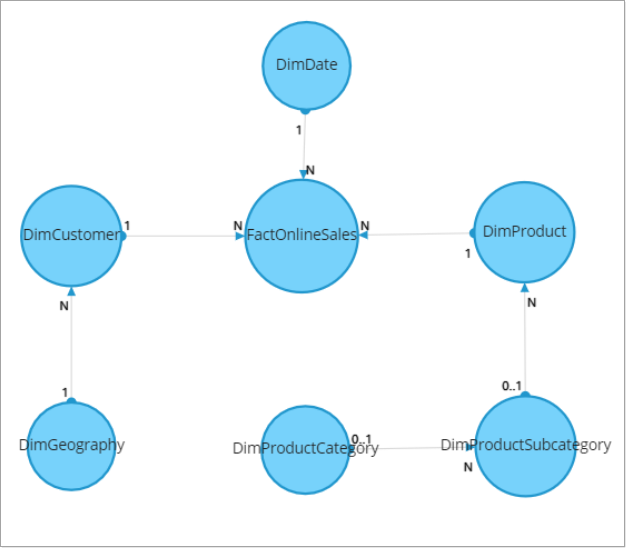Here you can add a measure for the 'FactOnlineSales' table to calculate the gross profit for online sales.

``````SUMX (
FactOnlineSales,
FactOnlineSales[SalesAmount] - FactOnlineSales[DiscountAmount] - FactOnlineSales[ReturnAmount] - FactOnlineSales[UnitCost] * ( FactOnlineSales[SalesQuantity] - FactOnlineSales[ReturnQuantity] )
)``````

Similarly, you can add a calculated column for the 'DimCustomer' table to group the customers based on their salary income.

``````SWITCH (
TRUE,
DimCustomer[YearlyIncome] < 50000, "Low",
DimCustomer[YearlyIncome] >= 50000 && DimCustomer[YearlyIncome] < 100000, "Medium",
DimCustomer[YearlyIncome] >= 100000, "High"
)``````

### Salient Points

1. The expressions for data model measures and calculated columns cannot be edited in the dashboard designer.

2. Data model measures and calculated columns cannot be removed or deleted in the dashboard designer.

3. The measures and calculated columns created in data models and dashboards are represented with different symbols, as shown in the table below.

Symbol

Data Attribute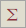Measure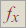Calculated Column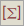Data Model Measure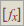Data Model Calculated Column